# Model differences

There are a variety of popular open-source libraries that provide some form of calibration. Each of these provides certain types of projection, distortion, and affinity-like models. However, these are not always the same.

If you're transitioning to TVCal and using Plexes, these differences may seem subtle and of no consequence. Unfortunately, there can exist a number of differences between models even when the models are expressed using the same parameters. Differences in how the models are posed may ultimately change how one leverages them in practice. Let's dive into the differences between Tangram Vision's models and some of the models used by popular open-source projects today.

## Cameras

A first look at our pages on projection, distortion, and affinity will set the stage as a good primer on our calibration models and describe some of the immediately obvious differences.

### Projection & Affinity

The most immediate difference between the model used by Tangram Vision and almost all popular computer vision models is our use of a single focal length in our pinhole projection model. Some notion of $$f_x$$ and $$f_y$$ is common in computer vision applications, but instead we opt to model using a single focal parameter $$f$$.

Often, the use of two focal lengths in the pinhole projection model is an attempt to model scale differences between the x and y-axes. This is sometimes referred to as having "non-square pixels." In our blog post on the matter, we describe the history behind the problem of "non-square pixels," and how it is more often than not a result of clock-skew inconsistencies between the CCD clock and DAC.

Regardless of the origin of the effect, it can still be observed. Consequently, we often want to model it. Instead of modeling this effect as two separate focal lengths, we instead model it as an affinity correction about the x-axis:

$g_{\mathsf{affinity}}(x) = \begin{bmatrix} a_1 (x - c_x) \\ 0 \end{bmatrix}$

which combined with pinhole projection produces the following model:

$\begin{bmatrix} x \\ y \end{bmatrix} = f \begin{bmatrix} X_c/Z_c \\ Y_c/Z_c \end{bmatrix} + \begin{bmatrix} c_x \\ c_y \end{bmatrix} + \begin{bmatrix} a_1 (x - c_x) \\ 0 \end{bmatrix}$

Notice that our affinity parameter $$a_1$$ is parameterized relative to our image coordinate $$x$$, not the object space $$f X_c / Z_c$$. This is done for the following reasons:

1. The affinity correction is purely modeling an aspect of image space. There are not, after all, different scales for meters or feet or inches or whatever depending on whether you measure in the x or y-direction when we define our target field.
2. This de-couples our estimation of the model parameter $$a_1$$ from our model parameter $$f$$.

The latter point is the most important one here. If we are to model this effect in terms of object space, we would instead have a model similar to the following:

$\begin{bmatrix} x \\ y \end{bmatrix} = f \begin{bmatrix} (1 + a_1) X_c/Z_c \\ Y_c/Z_c \end{bmatrix} + \begin{bmatrix} c_x \\ c_y \end{bmatrix}$

This form is quite compact, so let's expand it somewhat:

$\begin{bmatrix} x \\ y \end{bmatrix} = \begin{bmatrix} f \cdot X_c/Z_c \\ f \cdot Y_c/Z_c \end{bmatrix} + \begin{bmatrix} f \cdot a_1 \cdot X_c/Z_c \\ 0 \end{bmatrix} + \begin{bmatrix} c_x \\ c_y \end{bmatrix}$

The important part of this model to take note of is $$f \cdot a_1 \cdot X_c / Z_c$$. When estimating $$a_1$$ here, we are immediately correlating it with $$f$$, which is a difficult parameter to estimate. Not only does this mean that we have a strong projective compensation between $$a_1$$ and $$f$$, we also introduce a strong data dependency on our target field, since $$a_1$$ and $$f$$ here are going to necessarily have a strong projective compensation with $$X_c / Z_c$$ as well. This model makes it significantly more difficult to produce a robust and consistent calibration.

#### Other Projection Models

Other Projection Models

At present, Tangram Vision only supports pinhole projection models. If you're interested in other forms of projection (orthographic, equidistant, dual-sphere, etc.) contact us and let us know! Doing so will help us prioritize which models to add next.

### Distortion

Tangram Vision presently supports two kinds of distortion intrinsics — Brown-Conrady $$(k_1, k_2, k_3, p_1, p_2)$$ distortions and Kannala-Brandt $$(k_1, k_2, k_3, k_4)$$ distortions. While there are some differences between these two models, the points below apply equally to both types of distortions.

#### OpenCV: Undistorted to Distorted

One the first major differences to consider is what "distortion" we're modeling. Open-source libraries such as OpenCV will model distortion as an additive effect in object space. First, lets look at the general geometry of the problem as follows: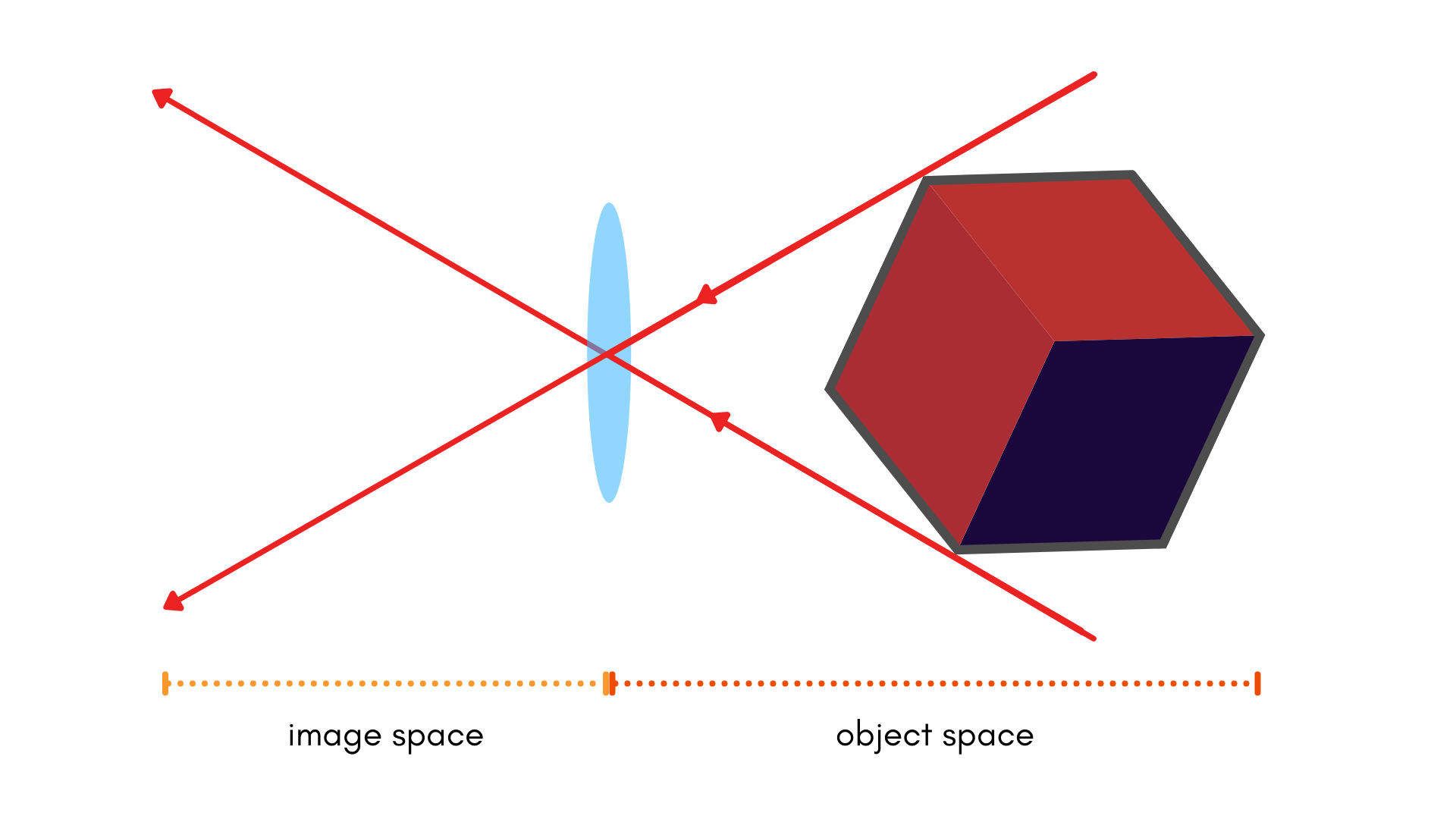In a pinhole model, distortion appears as if it were a "shift" in the final image coordinates. In the above image, we can visualize radial lens distortion (and the associated "shift") as the orange area. In this initial photo, there is no distortion, because the lines going into our lens (treated as a pinhole) and the lines coming out the other side are entirely straight. Let's look at what this might look like with distortion: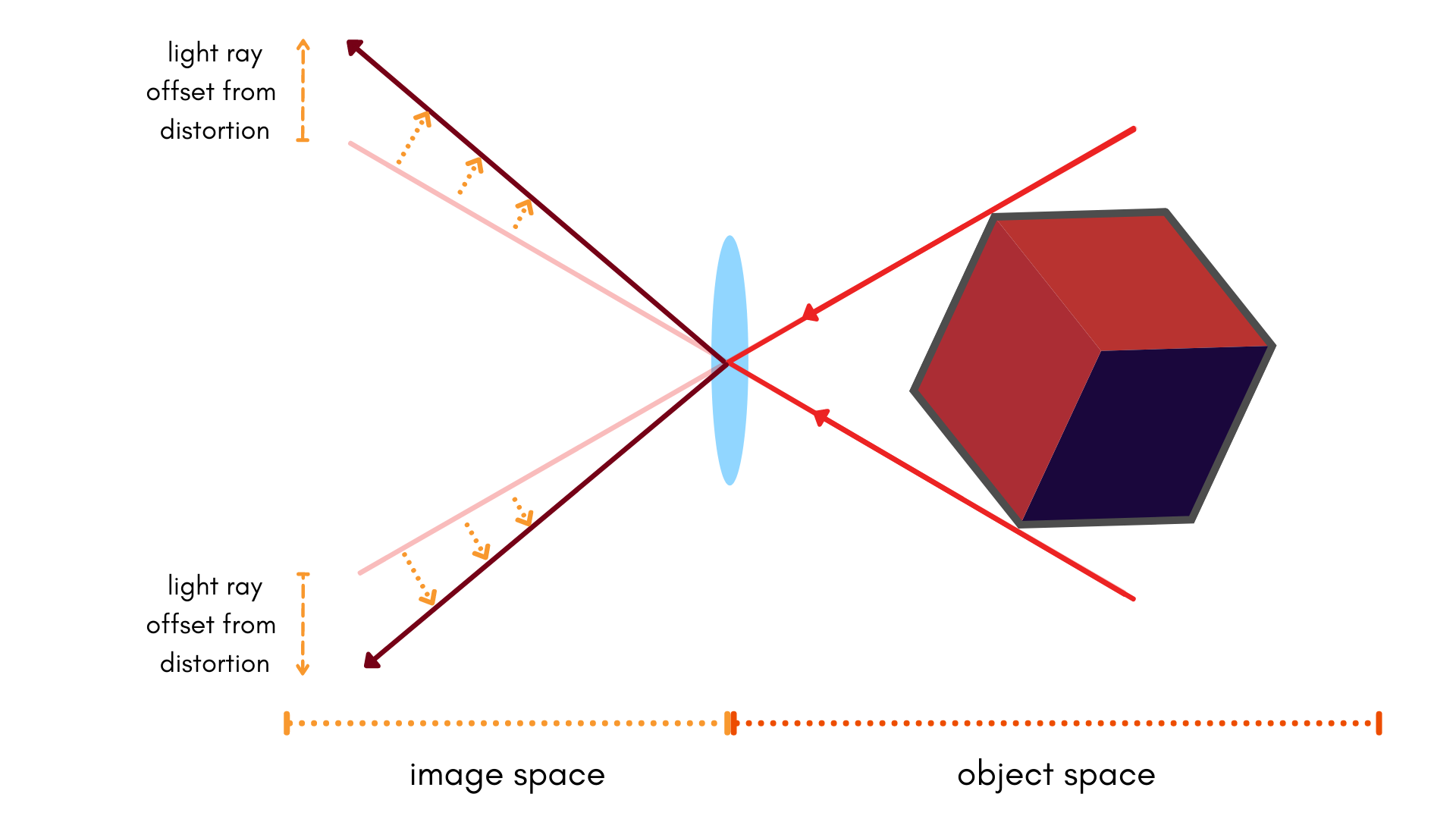In this image the dotted lines are in the same position they were in the original image. However, the projected position of a point in the image space (left side of the lens) is shifted. This is the effect of distortion.

Now we consider how this is modeled in OpenCV. In OpenCV, distortion is "added" to the perfect scenario. It is parameterized in terms of a change of our object space: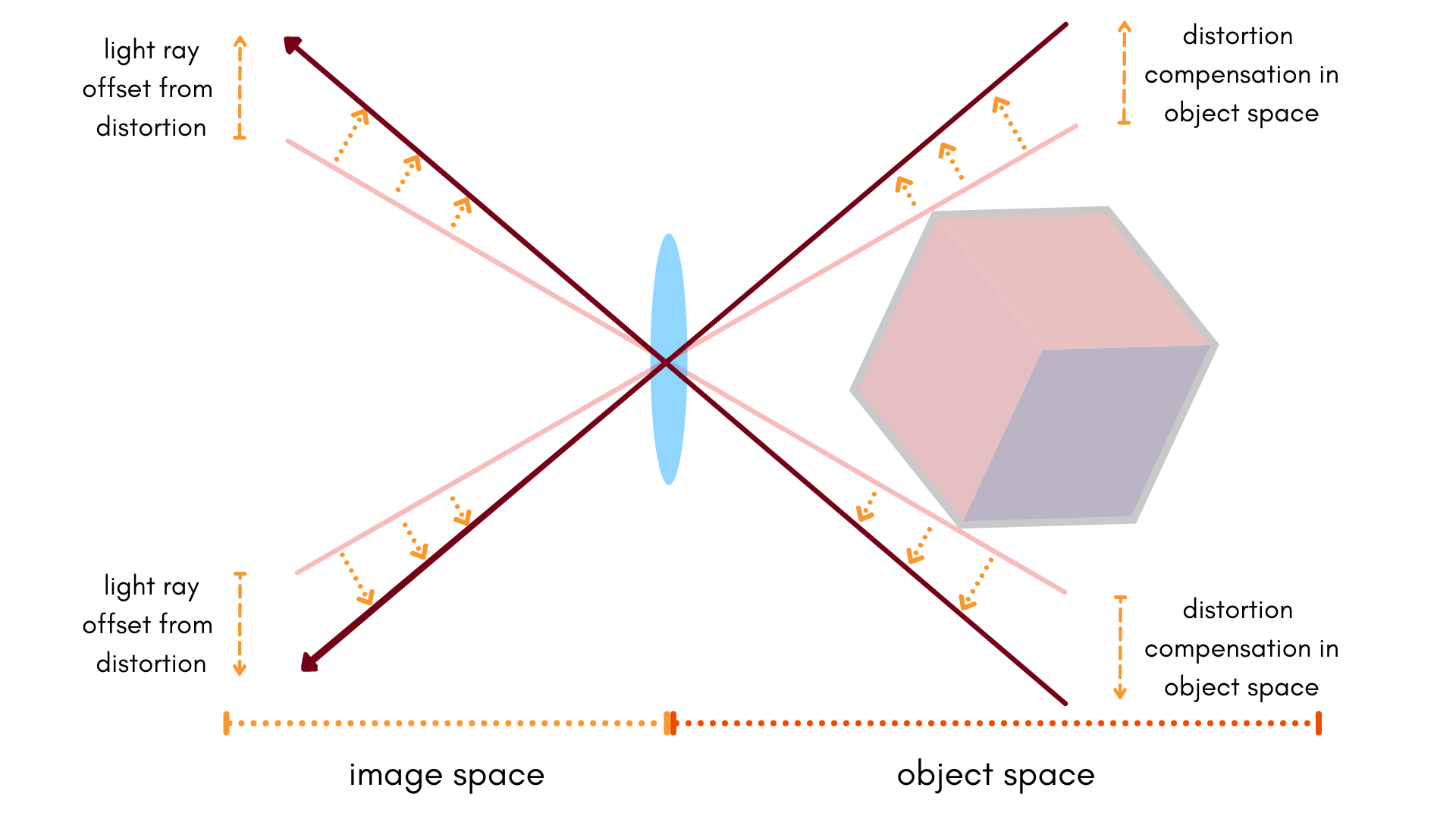So one can see, the pinhole model is preserved and the lines are thus straight again. From a mathematical point of view, this effectively means that distortions are parameterized by the object space. For Brown-Conrady distortion, this takes the form of:

$U = \frac{X_c}{Z_c}$
$V = \frac{Y_c}{Z_c}$
$r = \sqrt{U^2 + V^2}$
$\begin{bmatrix} x \\ y \end{bmatrix} = f \begin{bmatrix} X_c / Z_c \cdot (1 + k_1 r^2 + k_2 r^4 + k_3 r^6) + 2 p_1 U V + p_2 (r^2 + 2 U^2) \\ Y_c / Z_c \cdot (1 + k_1 r^2 + k_2 r^4 + k_3 r^6) + p_1 (r^2 + 2 V^2) + 2 p_2 U V \end{bmatrix} + \begin{bmatrix} c_x \\ c_y \end{bmatrix}$

As was the case with affinity, parameterizing in terms of the object space parameters conflates these parameters with the focal length. This model, which is not what Tangram Vision uses, is a function that maps undistorted points to distorted points.

This has a number of key disadvantages:

1. As mentioned previously, this conflates the determination of the parameters with the determination of the focal length. This is one kind of projective compensation.
2. Once determined, these parameters take the true location of a point within an image (in other words, an undistorted point), and determine the shift that would occur on that point due to distortion. One can take the true location of points (if known), and then distort these points. But that's not typically what we want! When we capture images from a camera, distortion has already occured due to the light passing through the lens. So we don't have the "true" or undistorted location of the observed point, instead the point we have observed has already been distorted (by the lens!).

#### Tangram Vision: Distorted to Undistorted

In direct contrast to software like OpenCV, Tangram Vision models distortion in image space.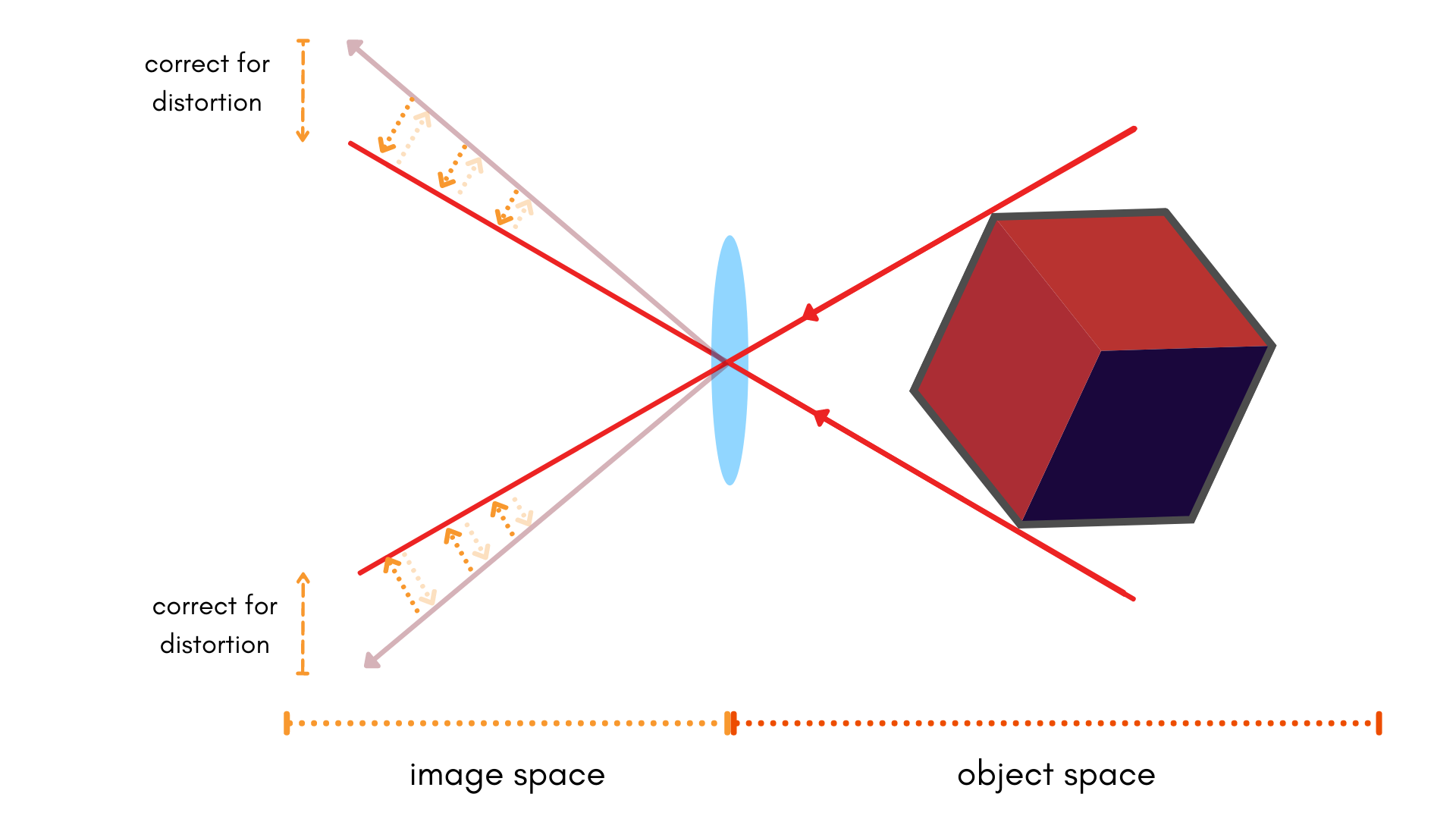In this model, the distorted point locations are what's observable, but the solid lines are the true location. Instead of adding distortion to the object space, the Tangram Vision Platform optimizes for a correction in image space. This takes the following form mathematically:

$u = \left(x - c_x\right)$
$v = \left(y - c_y\right)$
$r = \sqrt{u^2 + v^2}$
$\begin{bmatrix} x \\ y \end{bmatrix} = f \begin{bmatrix} X_c / Z_c \\ Y_c / Z_c \end{bmatrix} + \begin{bmatrix} c_x \\ c_y \end{bmatrix} + \begin{bmatrix} u (k_1 r^2 + k_2 r^4 + k_3 r^6) + p_1 (r^2 + 2 u^2) + 2 p_2 u v \\ v (k_1 r^2 + k_2 r^4 + k_3 r^6) + 2 p_1 u v + p_2 (r^2 + 2v^2) \end{bmatrix}$

In this way, rather than modeling distortion as being added to an image (and later undistorting it), we are instead modeling a correction. This is sometimes called the inverse Brown-Conrady model. Intel® RealSense™ even refers to it as such for select models of their cameras in their documentation. In our API, we avoid the inverse terminology for both Brown-Conrady and Kannala-Brandt, but you can expect that both models are constructed in this way.

This model has the advantage of behaving as a function that maps distorted points in a frame directly to the "true" locations of those points, in closed form. This is more commonly what we want when measuring distortion, rather than adding distortion to our object space to compensate for the adjusted positions of distorted points in an image.

In addition, these parameters are computed in image space, which makes them independent of other parameters being solved for (excepting $$c_x$$ and $$c_y$$). This does mean that we often have to be careful of projective compensation between $$c_x$$ and $$c_y$$, but by and large this can be mitigated by capturing data with different orientations. See our tutorial on this for more information.

In most cases, you'll just want to apply the above parameters directly to the point coordinates in an image directly to map distorted points from a raw camera frame to undistorted point coordinates. If you are looking to map a point in 3D space and project this back to a (distorted) pixel location, you'll need to generate some form of lookup table or iterative process to do so.

#### Balanced vs. Gaussian Profile

Some software opts to use the "Balanced" distortion profile. A distortion profile that is balanced (as opposed to the normal, Gaussian profile) will ultimately optimize to the same degree of reprojection error, but balances the distortion by adjusting the profile of the distortion curve (the polynomial described by your distortion parameters). This balancing in effect will limit the maximum amount of distortion at a given $$r$$ or $$\theta$$ within the image (whether you're using the balanced Brown-Conrady or Kannala-Brandt distortions).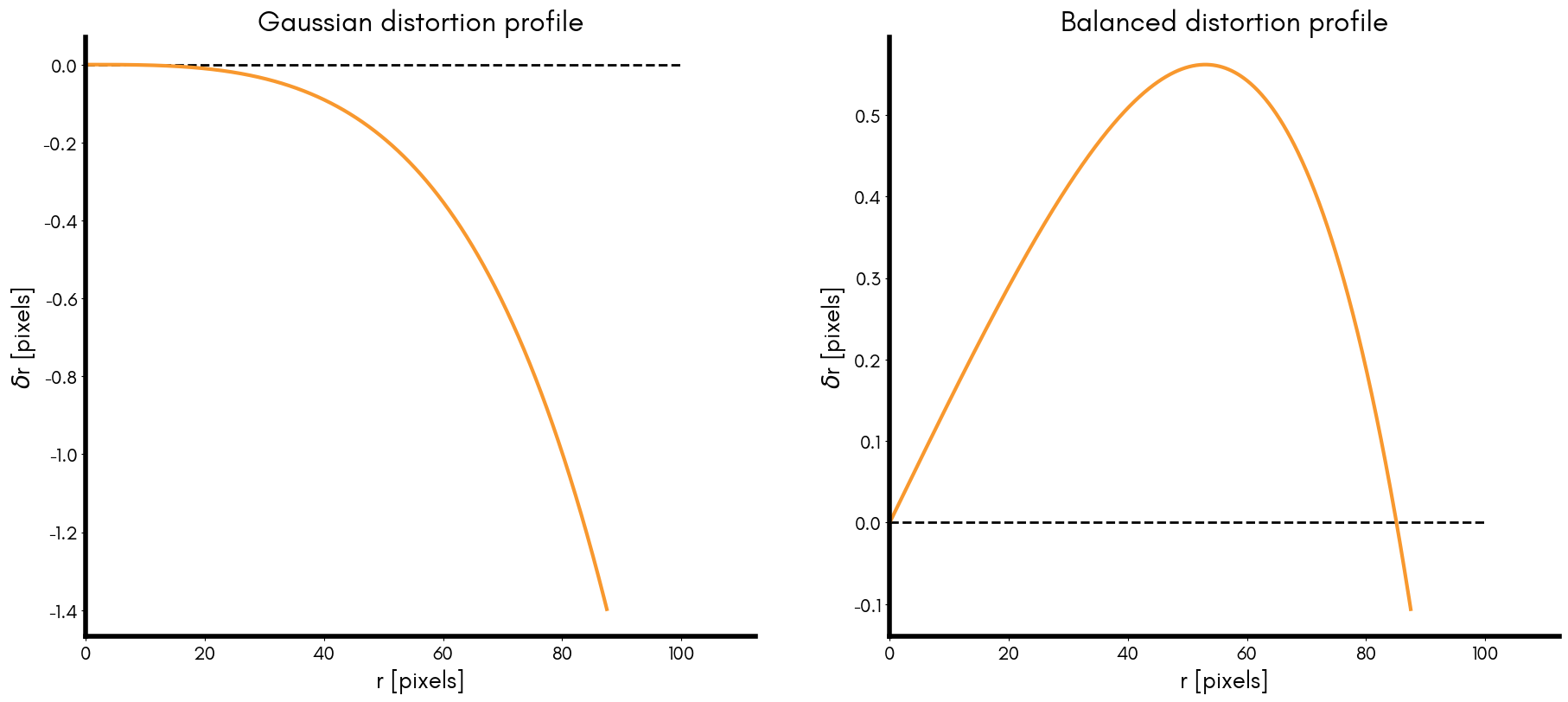The above graphs were generated to demonstrate what the distortion curve looks like relative to the radius for purely radial distortion (Brown-Conrady). On the left hand side we have the Gaussian profile, which is what we refer to as the "normalized" distortion. On the right, we have the Balanced profile, which is balanced such that the distortion is zero at a radius of ~85 pixels.

So although these two distortion profiles can produce the same reprojection errors, how are two different sets of distortions equivalent for the same camera? Well, this balancing is achieved by altering the focal length or principal distance, resulting in a new virtual focal length. Specifically, it removes the linear trend from the Gaussian profile through this focal length alteration. In the example above, the focal length is scaled by about 1.5% to produce a new virtual focal length and balanced distortion curve from the same distortion parameters.

Note

Sometimes the "virtual focal length" is referred to as a calibrated principal distance, which muddies up the terminology considerably. We've avoided calling it that here because it is easy to confuse the concept of a virtual focal length with a focal length that's actually been derived through a calibration process!

The balanced distortion profile does not provide any advantage to the calibration process. Historically, this technique was used to set the upper numerical limit on distortion observed from mechanical stereo-plotters, which had physical limits constraining how much distortion could be observed. This was a great aide for users of these mechanical devices, but no longer makes sense if we're applying distortion parameters numerically with a computer.

The downsides of the balanced profile do exist, however:

1. If we're shifting the focal length to some "virtual" value, this effectively means that we're scaling focal length. An astute reader might realize that in cases where $$f_x$$ and $$f_y$$ are used, we are conflating that focal shift across both parameters. In the case of Tangram Vision's model, this would produce a correlation between our distortion parameters and focal length, as well as a correlation between the distortion parameters and affinity scale.
2. We have to make a choice about where we want to balance our distortion to. In the example above it balances such that distortion is zero at ~85 pixels. Is this correct for every camera? How do we pick a(n otherwise arbitrary) value to balance towards?

OpenCV does in fact still use a balanced profile in their fisheye model. In their documentation, they describe the distortion as:

\begin{align} g_{\mathsf{fisheye}}(\theta) &= \theta (1 + k_1 \theta^2 + k_2 \theta^4 + k_3 \theta^6 + k_4 \theta^8) \\ &= \theta + k_1 \theta^3 + k_2 \theta^5 + k_3 \theta^7 + k_4 \theta^9 \end{align}

This is fairly close to what is described by Kannala-Brandt:

\begin{align} g_{\mathsf{KB}}(\theta) &= k_1 \theta + k_2 \theta^3 + k_3 \theta^5 + k_4 \theta^7 + \ldots \end{align}

As can be seen, the linear component Kannala-Brandt formulation (seen as $$k_1$$ in $$g_{\mathsf{KB}}(\theta)$$) does not exist in the OpenCV fisheye formulation. This is the linear component of the Gaussian profile, which is set to 1 in the Balanced profile. Tangram Vision avoids this balancing due to the disadvantages listed previously. Just remember that when using our distortion models, that e.g. our $$k_1$$ through $$k_4$$ terms may be shifted compared to how OpenCV describes them.